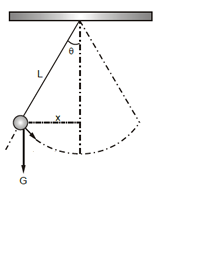# Maximum height reached and the maximum angle made by a simple pendulum Calculator

This CalcTown calculator calculates the maximum height reached and the maximum angle made by the bob of simple pendulum when given a velocity at its mean position.

At the highest point, the instantaneous velocity of the bob becomes zero.

m
m/s

#### Result

degree
m